International
Tables for
Crystallography
Volume C
Mathematical, physical and chemical tables
Edited by E. Prince

International Tables for Crystallography (2006). Vol. C, ch. 6.2, p. 598

## Section 6.2.6. Some remarks about the integrated reflection power ratio formulae for single-crystal slabs

H. Lipson,a J. I. Langforda and H.-C. Hub

aSchool of Physics & Astronomy, University of Birmingham, Birmingham B15 2TT, England, and bChina Institute of Atomic Energy, PO Box 275 (18), Beijing 102413, People's Republic of China

### 6.2.6. Some remarks about the integrated reflection power ratio formulae for single-crystal slabs

| top | pdf |

The transfer equations for intensity may be rewritten in the form of one-dimensional power transfer equations (Hu & Fang, 1993). The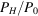in (b) and (c) for a mosaic crystal slab under symmetrical and unsymmetrical Bragg and Laue geometries are the general solutions of power transfer equations employing three dimensionless parameters b, ξ and τ. For a crystal slab with a rectangular mosaic distribution, considering multiple reflection, the integrated reflection power ratio, ρ′, can be obtained by substituting σ0 for σ in the formulae forand multiplying the result by the mosaic width. However, for crystals with other kinds of mosaic distribution, the corresponding ρ′ can be obtained only by integrating the expression for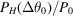over the whole range of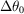. Formulae (1)–(3) listed in Table 6.3.3.1, i.e. the transmission coefficient A multiplied by Q, QA, are identical to those of (b) and (c) for the case of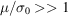, which is the integrated reflection power ratio for a crystal slab based on the kinematic approximation without consideration of multiple reflection.

The secondary extinction factor for X-ray or neutron diffraction in a mosaic crystal slab can be obtained as ρ′/(QA), in which the integrated reflection power ratio with consideration of multiple reflections can be obtained as described above.

Both the transmission power ratio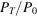and the absorption power ratio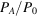can also be obtained by solving the power transfer equations. For details, see Hu (1997a,b), Werner & Arrott (1965) and Werner, Arrott, King & Kendrick (1966).

### References

Hu, H.-C. (1997b). A universal treatment of X-ray and neutron diffraction in crystals. II. Extinction. Acta Cryst. A53, 493–504.
Hu, H.-C. & Fang, Y. (1993). Neutron diffraction in flat and bent mosaic crystals for asymmetric geometry. J. Appl. Cryst. 26, 251–257.
Werner, S. A. & Arrott, A. (1965). Propagation of Bragg-reflected neutrons in large mosaic crystals and the efficiency of monochromators. Phys. Rev. 140, A675–A686.
Werner, S. A., Arrott, A., King, J. S. & Kendrick, H. (1966). Propagation of Bragg-reflected neutrons in bounded mosaic crystals. J. Appl. Phys. 37, 2343–2350.